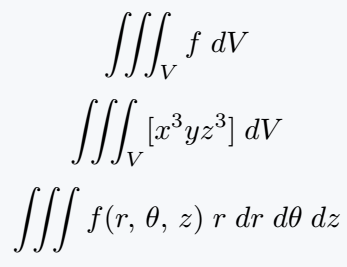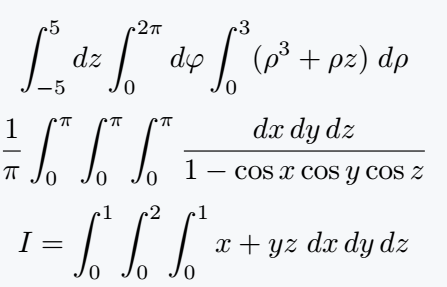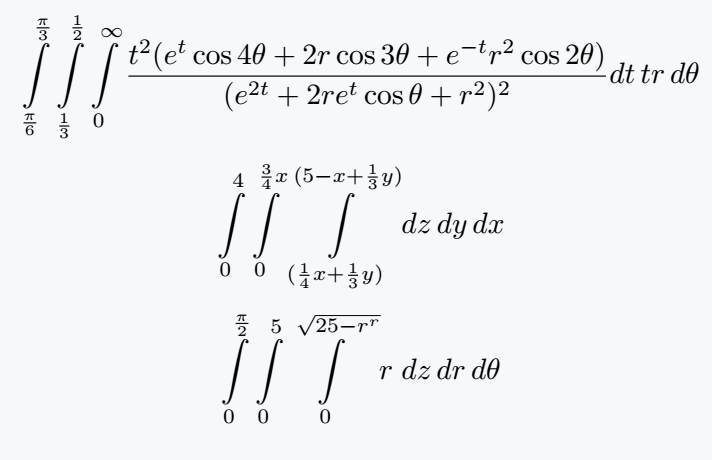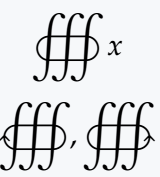# How to write triple integral(∭) and volume integral(∰) in LaTeX?

Hearing the name of Triple Integral, you can understand that this symbol is made by sitting side by side with three single Integral symbols.

When you use without limit or lower limit, just use \iiint command is enough.

\documentclass{article}
\usepackage{amsmath}
\begin{document}
$\iiint_V f\;dV$
$\iiint_V [x^3 yz^3]\;dV$
$\iiint f(r,\,\theta,\,z)\;r\;dr\;d\theta\;dz$
\end{document}

Output :Even if the single integral symbol is in the default, you need to use the package for the Triple Integral symbol.

## Triple integral symbol with limits

Cannot pass the limit inside Direct Triple Integral. For this, you need to use Single Integral three times with limit.

\documentclass{article}
\begin{document}
$\int^5_{-5}dz\int^{2\pi}_0 d\varphi\int^3_0 (\rho^3 +\rho z)\;d\rho$
$\frac{1}{\pi}\int^\pi_0\int^\pi_0\int^\pi_0\frac{dx\,dy\,dz}{1-\cos x\cos y\cos z}$
$I=\int^1_0\int^2_0\int^1_0 x+yz\; dx\,dy\,dz$
\end{document}

Output :If you want, you can create a command with six arguments in which six limits will be passed.

\documentclass{article}
\newcommand{\tplint}{\int\limits^{#1}_{#2}\int\limits^{#3}_{#4}\int\limits^{#5}_{#6}}
\begin{document}
% Using \limits for better output and \newcommand for easy to write
$\tplint{\frac{\pi}{3}}{\frac{\pi}{6}}{\frac{1}{2}}{\frac{1}{3}}{\infty}{0}\frac{t^2(e^t\cos 4\theta +2r\cos 3\theta +e^{-t}r^2\cos 2\theta)}{(e^{2t}+2re^t\cos\theta +r^2)^2}dt\,tr\,d\theta$
$\tplint{4}{0}{\frac{3}{4}x}{0}{(5-x+\frac{1}{3}y)}{(\frac{1}{4}x+\frac{1}{3}y)}dz\,dy\,dx$
$\tplint{\frac{\pi}{2}}{0}{5}{0}{\sqrt{25-r^2}}{0}r\;dz\,dr\,d\theta$
\end{document}

Output :When using an integral symbol always remember a usage, when a limit is used completely above and below the integral symbol, then the \limits command is required.

## Triple close integral(volume integral) symbol in LaTeX

With the \oiiint command, you can represent the volume integral symbol. Of course, you will need a package for this.

\documentclass{article}
\usepackage{pxfonts}
\begin{document}
$\oiiint x$
$\oiiintclockwise,\oiiintctrclockwise$
\end{document}

Output :#### Md Jidan Mondal

LaTeX expert with over 10 years of experience in document preparation and typesetting. Specializes in creating professional documents, reports, and presentations using LaTeX.